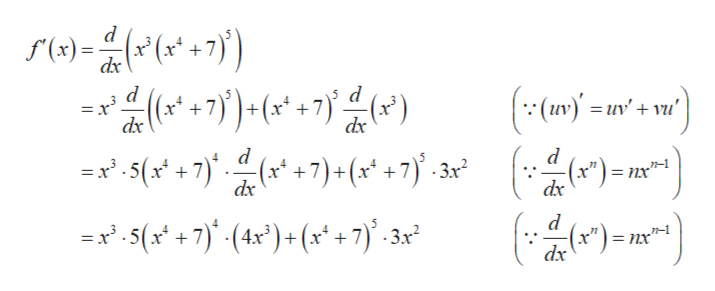Question
24 views
Find f'(x) for f(x)= x3(x4+7)5

check_circle

Step 1

Obtain the derivative of f(x...help_outlineImage Transcriptionclosed ()=(+7) dx ((I)In (x 7 dx dx d d - (x*) 7)+ (x* + 7) . 3x? (ax) (x")= x5(x +7 +7)+(x + 7)-3x2 nx пх" d x.5(x* +7) (4x*)+ (x* +7} . 3x n-1 3r2 = nx dx fullscreen

### Want to see the full answer?

See Solution

#### Want to see this answer and more?

Solutions are written by subject experts who are available 24/7. Questions are typically answered within 1 hour.*

See Solution
*Response times may vary by subject and question.
Tagged in

### Derivative